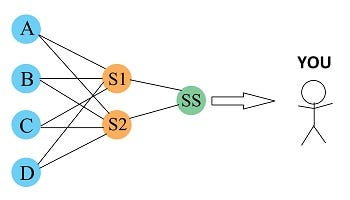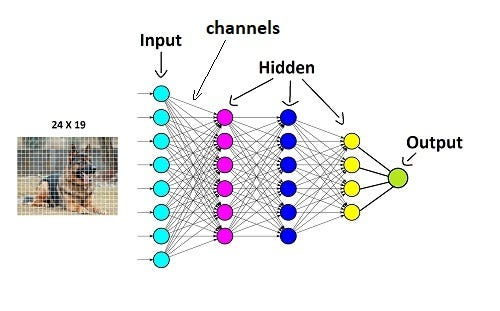Beginners guide to Neural Networks (understanding neural networks as simple as possible)

A neural network is a network of neurons inside our brain and an artificial neural network is inspired by our brain where all the nodes(neurons) are interconnected to form a complex network. Don't worry if you didn't get it, I will try to explain neural networks as simple as possible without using any complex math.Image by Ermal Tahiri from Pixabay

Let’s assume there is a group of people who have never seen a dalmatian dog which is a breed of dog. Your task is to train them to identify the dalmatian dog among other dog breeds.

You divide them into different team and assign them different task. Like, team A has to identify the eyes of dog, team B has to identify ears, team C to identify nose team D to identify nose. So, each of these team are working on detecting a specific part of dog’s body from an image. The way they give their decision is by using a score of 0 to 1, where 0 means this is definitely not a dalmatian eyes, 0.4 means not sure maybe 50 50 and 1 means it is definitely dalmatian's eyes. You pass an image of dalmation dog to these teams. These teams makes random guess and pass there score to their superior S1 and S2.

S1 and S2 use the information provide by different teams and use a formula to calculate their score. You can think of formula like team A gives a of score 0.3 for eyes, team B give score of 0.5 for ears similarly team C and D give score of 0.33 and 0.25 so formula will become

dog = (eyes x 0.2 + ears x 0.5 + legs x 0.3 + nose x 0.22), here 0.2, 0.5, 0.3, 0.22 are importance given to the different parts of dog. so final score will be

dog = (0.3x0.2 + 0.5x0.5 + 0.33x0.3 + 0.25x0.22) = 0.76

Any score above 0.5 means it is a dalmatian dog and any score below 0.5 means not a dalmatian dog.

Now S1 and S2 calculate their scores like above and pass their score to their superior SS.

Now SS will take decision based on the score values and finally came to you and tell “this is not a dalmatian dog”, but you know that it’s a dalmation dog. so, you reply, “it is a dalmatian dog, go correct the mistake”.SS giving output to you

Then SS goes back to the S1 and S2 and tell them there is a mistake, then S1 and S2 pass this feedback to the team A, B, C and D and tell them to be careful next time. Using this feedback, they adjust the weights to calculate score and try to give better results next time. You can take n number of dog’s images and repeat the same process. So, the same process is repeated where teams make a random guess then superior S1 and S2 calculate their score and goes to SS and SS tells you that this is dalmatian or not, you will tell SS if the answer is correct or not and the error feedback is passed on to rest of the team and the team keeps and keep on adjusting their weights or adjusting their brains in a way that they can finally come up with the right answer. You can give thousands of dog images and after training this team for so many images eventually the team becomes better at dalmatian dog detection. Initially it will make a lot of errors but as the time goes it will keep on improving.

In the above example, all the teams, their superiors and SS all are neurons. Team A, B, C, D are in the input layer and superior S1 and S2 are in the hidden layers and SS is the output layer.

In the real life you don’t need to give different task to each neuron, when you are dealing with complex data sets you do not know what features you are looking at. So, you just build a neural network which has input layer couple of hidden layers and the output layer and neural network will figure out for you. So, each individual neuron will figure out what tasks it needs to work on, all you need to do is to feed a lot of data to it.

The motivation behind neural networks came from the way human brain works. If you remember those days when as a kid you were trying to learn bicycle you would initially fall down, then you try again and eventually you master that skill. During those trial and errors what’s happening is our brain has billions of neurons and in those neurons this training process is constantly going on so, there is an error loop or a back propagation feedback that goes inside those tiny neurons and the weights between those neurons are constantly being adjusted until you get into a situation where you are making minimum amount of mistake.

Now, let’s see how neural networks works in machine learning.

Neural networks are made up of layers of neurons. These neurons are the core processing units of the network. First we have the input layer which receives the input, then the hidden layers which perform most of the computations required by our network and then the output layer which predicts our final output.

Let’s take our above problem. Here we have an image of a dog, imagine this image is composed of 24 by 19 pixels which make up for 456 pixels.

Each pixel is fed as input to each neuron of the first layer. Neurons of one layer are connected to neurons of the next layer through channels.A Simple Neural Network

Each of these channels is assigned a numerical value known as weight the inputs are multiplied to the corresponding weights and their sum is sent as input to the neurons in the hidden layer. Each of these neurons is associated with a numerical value called the bias which is then added to the input sum. This value is then passed through a threshold function called the activation function. The result of the activation function determines if the particular neuron will get activated or not. An activated neuron transmits data to the neurons of the next layer over the channels. In this manner the data is propagated through the network this is called forward propagation. In the output layer the neuron with the highest value fires and determines the output. The values are basically a probability.

Now assume that our neural network makes wrong prediction, how does the network figure this out? Note that our network is yet to be trained. During this training process along with the input our network also uses the output fed to it. The predicted output is compared against the actual output to realize the error in prediction. The magnitude of the error indicates how wrong we are and the sign suggests if our predicted values are higher or lower than expected. The arrows here give an indication of the direction and magnitude of change to reduce the error. This information is then transferred backward through our network this is known as back propagation. Now based on this information the weights are adjusted this cycle of forward propagation and back propagation is iteratively performed with multiple inputs. This process continues until our weights are assigned such that the network can predict the shapes correctly in most of the cases. This brings our training process to an end you might wonder, how long this training process takes? Neural networks may take hours or even months to train depending on the size of the data and computation power.

I hope now you have got a good understanding of neural networks. Don’t worry about the fancy terms like “activation function”, we will discuss them in upcoming articles.

Watch this video and learn how pooling layer works in CNN.

Check out my other article on “mean average precision for object detection”, where I explained how mAP is calculated for objection detection using python.

Understanding mean Average Precision for Object Detection (with Python Code)

Photo by  Avel Chuklanov  on  Unsplash If you ever worked on object detection problem where you need to predict the bounding box coordinates of the objects, you may have come across the term mAP (mean average precision). mAP is a metric used for evaluating object detectors. As the name suggest it is the average of the AP. To understand mAP , first we need to understand what is precision, recall and IoU(Intersection over union). Almost everyone is familiar with first two terms, in case you don’t know these terms I am here to help you. Precision and Recall Precision: It tells us how accurate is our predictions or proportion of data points that our model says relevant are actually relevant. Formula for precision Recall: It is ability of a model to find all the data points of inte